# Mathematics - All about Complex numbersHello it's a me again drifter1! Today we continue with Mathematics to talk about everything that you have to know about Complex numbers. I will give you all the theory with examples of this topic of maths. This knowledge will be enough in anything that needs Complex numbers in general. I hope that this post will be useful!

For printing equations I will use the tool quicklatex which was recommended by @drdawud.

So, without further do, let's get started!

## Getting into the need of Imaginary numbers

When solving equations in Mathematics we sometimes get in trouble, cause no real number solution(s) can be found.

This means that we have:

• no natural (0, 1, 2, ...)
• no integer(..., -2, -1, 0, 1, 2, ...)
• no rational (ratio's of integers)
• no real (numbers with decimals / digits after the decimal point) AND
• no irrational (reals that are not rational) solutionTo find the solution of such a 2nd-order polynomial we use the formula:The subroot is negative and so we say that the equation has no solution. But, we are wrong!

The right thing to say is : "There is no solution in the real number set".

We know that in general the solutions depend on the subroot:

• b^2 - 4ac > 0 => 2 solutions
• b^2  - 4ac = 0 => 1 double-solution
• b^2 - 4ac < 0 => no real solutions

Let's stay on that last line: "there are no real solutions".

We know that a polynomial equation of 2nd-order has 2 solutions.

For positive we have 2 solutions, for zero we have 1 double-solution and so the same solution twice, but either way they are 2.

Does this mean that there are 2 "hidden" solutions in the last case? Yes it does!

## The Complex number set

But, where are those solutions at? Well, they are in an extension of the real number set: the complex number set.

Because, the problem of finding the solutions is in the negative subroot we define a number i, the so called imaginary unit or indeterminate that will help us solve it.

i is a imaginary number with the following property: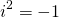Using it in the root of our previous example now gives us: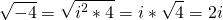So, the solutions are:There it is! There are really 2 solutions for such equations that you could not solve before!

These solutions are not real numbers, but the problem was not finding real solutions, but finding 2 solutions that we knew existed, because of the polynomial equation's order that is 2!

Thinking about this formula and seeing the form of the solutions we can clearly see how an complex number is build up!

A complex number is of the form: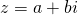where:

• z is the complex number notation
• a and b are real numbers
• i is the imaginary unity

## Complex number properties

Some more things about z = a + bi:

• a is called the real part and is also noted as Re(z)
• b is called the imaginary part and is also noted as Im(z)
• if b = 0 then z is of course a real number
• if a = 0 then z is a "purely" imaginary number

The set of "purely" imaginary numbers is noted as I.

Because when setting b = 0 we get real numbers we say that:

• the complex number set C of course contains the real number set R, with R being a subset of C
• all the properties of addition and multiplication of R apply to C too
• if z is a complex number then: z+0 = z and z*1 = z
• Each complex number z can be written as: z = a + bi, where a and b are unique

The thing that C doesn't inherit from R is the arrangement and order relations of numbers.

We can't say that:cause then -1 > 0, which can't be true!

Of course 2 complex numbers z = a + bi and w = c + di are equal if:

1. a = b
2. c = d

Of course this means that z = 0 only if a + bi = 0 => a = b = 0

For this last equation we of course have to note that a AND b are BOTH real numbers!

## Cartesian form/notation of Complex numbers

Each complex number is defined by the pair (a, b) = (Re(z), Im(z)).

Using the 2-dimensional Cartesian coordination system each complex number can so be represented by a point M(z) = M(a, b) on top of that complex plane, that contains a real(Re) and imaginary(Im) axis.Because of that representation we can now do calculations on complex numbers like they where vectors.

So, if z = (OA) and w = (OB)

where z, w are complex numbers and OA, OB their corresponding vectors

Then we can say that:

k*OA + λ*OB is a vector OP that corresponds to a new complex number v = kz + λw

We can clearly see that the addition of vectors is the same as the addition of the complex numbers directly!

## Operations between Complex numbers

if z1 = a + bi and z2 = c + di are two complex numbers then:If z = a + bi:Everytime i is in the denominator, like in the divisions of above, we multiply the numerator and denominator with the conjugate of the denominator (more about that later on). For z1/z2 with (c - di) and for 1/z with (a - bi).

This should be a rule for you, cause it is needed everytime we have i in the denominator!

The addition in "vector-form" looks like this: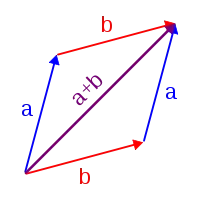Of course because a complex number can be represented by vectors we have that:

• the vector z1 + z2 is the addition of the vectors z1 and z2
• the vector z1 - z2 is the addition of the vectors z1 and -z2 (or the substraction with z2)
• etc.

## Exponentials of Complex numbers

Suppose z = a + bi is a complex number.

The powers z^k (with k being natural) are:For the last one k-times.

Of course: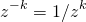if z is not zero!

For the powers of integer exponents the same properties and rules apply as in real numbers.

Some properties of rational exponents can be defined, but there are also some exceptions.

This means that we will just remember that we can have integer exponents and use them like real numbers!

What about the powers of i?

Supposing n = 4q + r we have that r = {0, 1, 2, 3}.

And so: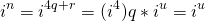Which means that we end up with the cases:For example: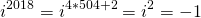## Conjugate of Complex numbers and Properties

The complex number z* (or z with line on top) that has the same real part (a) but the opposite in sign imaginary part (b) as z is called the conjugate of z.

For example conjugate of z = 2 - 3i is z* = 2 + 3i.

The geometric represenation of the conjugate is:Geometrically we can see that the conjugate of z (z*) and z are symmetrical to the x'x axis.

Which means that if z = a + bi, which is the point M(z) = (a, b) then:

z* = a - bi , which is the point M(z*) = (a, -b)

The following are some of the most important properties of the conjugate:

• zz* = (a + bi)(a - bi) = a^2 + b^2
• (z1 + z2)* = z1* + z2*, which can be generalized to n numbers, cause we apply the conjugate for each term/number individually
• (z1z2)* = z1*z2*, which can again be generalized for n numbers.
• (z^n)* = (z*)^n, for every natural n>=0 [so the exponent can be put out of the conjugate]
• (z1/z2)* = z1*/z2*, so the conjugate can be applied to the numerator and denominator independently
• z + z* = 2a = 2Re(z) AND z - z* = 2bi = 2Im(z)i

From the last two properties we have that:

1. z is a real number if z* = z, cause z - z* = 0 and so there is no imaginary part.
2. z is a imaginary number if z* = -z, cause then z + z* = 0 and so there is no real part.

We use these exact properties to check if a complex number is real or imaginary!

## The equation az^2 + bz + c = 0, with a!=0

So, to sum up, let's get back to the equation we started with!

We have the equation: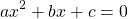where a, b, c are real numbers.

If x is in the real number set then it will also be a real number and so we have the results that we had before which are:

• b^2 - 4ac > 0 => 2 solutions
• b^2  - 4ac = 0 => 1 double-solution
• b^2 - 4ac < 0 => no real solutions

What if x is not a real number, but a complex number z?

Well, then:

• b^2 - 4ac > 0 => 2 real solutions
• b^2  - 4ac = 0 => 1 real double-solution
• b^2 - 4ac < 0 => 2 complex solutions which are supplementally (one is the conjugate of the other)

And we get our final solution from: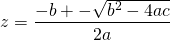Which will give us:for each of the 3 cases.

## Absolute value of Complex numbers and properties

Using the Cartesian form/notation for a complex number we can start talking about the absolute value of complex numbers.

The absolute value is defined as the distance of the origin point O(0, 0) and the point M(a, b) which represents the complex number in the complex plane.This means that the absolute value is also equal to the length of the vector OM.

In the Cartesian space this looks like this:So, the absolute value can be found using the Pythagorean Theorem on the triangle to find the diagonal.

That way the absolute value equals:If |z| = 0 then of course z = 0.

The following properties apply to the absolute value |z| of a complex number z:

• |z| = |-z| = |z*|, which means that the absolute value of z is equal to the absolute value of it's opposite and it's conjugate
• |z| = zz* [we already saw something similar before and this will of course be useful in calculations]
• |z1z2...zn| = |z1||z2|...|zn|, which means that the absolute value of an product of complex numbers can be written as the product of the absolute values of each complex number individually [simplest case is: |z1z2| = |z1||z2|]
• |z^n| = |z|^n for all naturals n >= 0, [the power can be found after finding the absolute value]
• |z1/z2| = |z1|/|z2|, the same way as in multiplication the division's absolute value equals the quotient of the absolute values of the numerator and denominator individually.

Worth noting is the relation: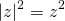Which is true for every real z, but not true for every complex number z

For example:but,and so: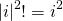But this makes it useful in another way, cause:

• If z is real then |z|^2 = z^2
• If z is imaginary then |z|^2 = -z^2

The previous statement and the last 2 properties of the conjugate (as I mentioned before) are both useful when checking if a complex number is real or imaginary! So, to check this we use either one of these, depending on the data we have given to us.

## Complex number argument and polar form

Using the Cartesian from again we can define another thing called the argument or phase of z.

The argument of z or arg(z) is the angle φ of (OM) where O(0, 0) is the origin point and M(a, b) is the point that represents the complex number z = a + bi.

This looks like this: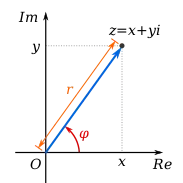A complex number z !=0 can of course have multiple arguments.

For two random arguments φ1, φ2 we have that:

φ2 - φ1 = 2kπ, with k being an integer and φ1, φ2 in radians

Because there are more then one arguments we use the principal argument in the interval [0, 2π) that defines the angle of (OM) to the x'x axis. That way:

Arg(z) = φ, where 0 <= φ < 2π

This one is of course unique.

So, if z != 0 is a complex number z = Re(z) + i*Im(z) then:

1. if Arg(z) = 0 <=> Im(z) = 0 and Re(z) > 0
2. if Arg(z) = φ, where 0 < φ < π/2 <=> tanφ = Im(z)/Re(z) with Im(z) > 0 or Re(z) > 0
3. if Arg(z) = π/2 <=> Re(z) = 0 and Im(w) > 0
4. If Arg(z) = φ, where π/2 < φ < π <=> tanφ = Im(z)/Re(z) with Im(z) > 0 or Re(z) < 0
5. If Arg(z) = π <=> Im(z) = 0 and Re(z) < 0

Using those cases and with a simple diagram we can solve any case!

This means that we end up with the following very useful cases for z = a + bi:

1. If z is real and a > 0 then Arg(z) = 0
2. If a = 0 and b > 0 then Arg(z) = π/2
3. If z is real and a < 0 then Arg(z) = π
4. If a = 0 and b < 0 then Arg(z) = 3π/2

The argument of the conjugate of z is:

Arg(z*) = 2π - φ

where Arg(z) = φ and φ in the interval (0, 2π)

## Trigonometric/Polar Form of complex numbers

Because of the argument and the Cartesian form we can also talk about the trigonometric or polar form of a complex number.

So, if z = a + bi is a complex number z != 0, M(a, b) is the point in the complex plane that represents it and arg(z) = φ is it's argument then:

a = |z|cosφ and b = |z|sinφ

Because of that:

z = |z|(cosφ + isinφ)

All this is true only if |z| > 0, which means that z != 0.

As mentioned previously the argument is not unique.

Suppose two complex numbers z1, z2 in polar form .

If z1 = |z1|(cos(φ1) + isin(φ1)) and z2 = |z2|(cos(φ2) + isin(φ2)

Then we can say that:

z1 = z2 <=> |z1| = |z2| AND φ1 - φ2 = 2kπ, where k is an integer

This can be useful when checking if two complex numbers are equal!

We can again define properties for operations that I will leave out for simplicity.

The polar form is useful when solving equations of complex numbers that are in the forms:

• z^n = 1
• z^n = a, where a is complex
• Polynomials of n-order/n-degree
• and more...

I will not get into how we solve them...

## Euler's formula

One very important formula is Euler's formula.

For any real number x we define: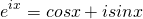I also wanted to do some exercises, but stepped on the following links that contain many many exercises that also contain things that we don't covered!

math-exercises

analyze math

### Image sources:And this is actually it and I hope that you learned something!

I also plan to make a post about trigonometry later on!

Bye!

H2
H3
H4
3 columns
2 columns
1 column